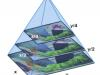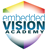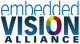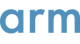DocumentsThe substantial parallel processing resources in modern GPUs makes them a natural choice for implementing vision-processing functions.This chapter describes how to re-use the code from this sample, the limitations of the test method, and a method of analyzing the results.This chapter describes the requirements needed to run this sample and the example hardware that produces the results in this guide.This chapter describes the conclusions from the optimization process.This chapter describes the performance of some common 3 x 3 convolution matrices using the fully optimized code.This chapter describes variations to the algorithm for 3 x 3 convolution matrices to create an algorithm for 5 x 5 convolution matrices.This chapter describes describes some general concepts to consider when optimizing kernels.This chapter describes describes some useful optimization methods, the logic for them, and the results they provide on the test platform.This chapter describes some initial (as well as the simplest and most intuitive) implementations of convolution algorithms.Convolution operations are important in image processing, particularly in filtering. GPU compute can improve performance significantly.